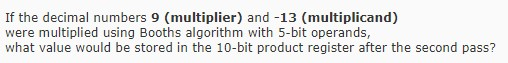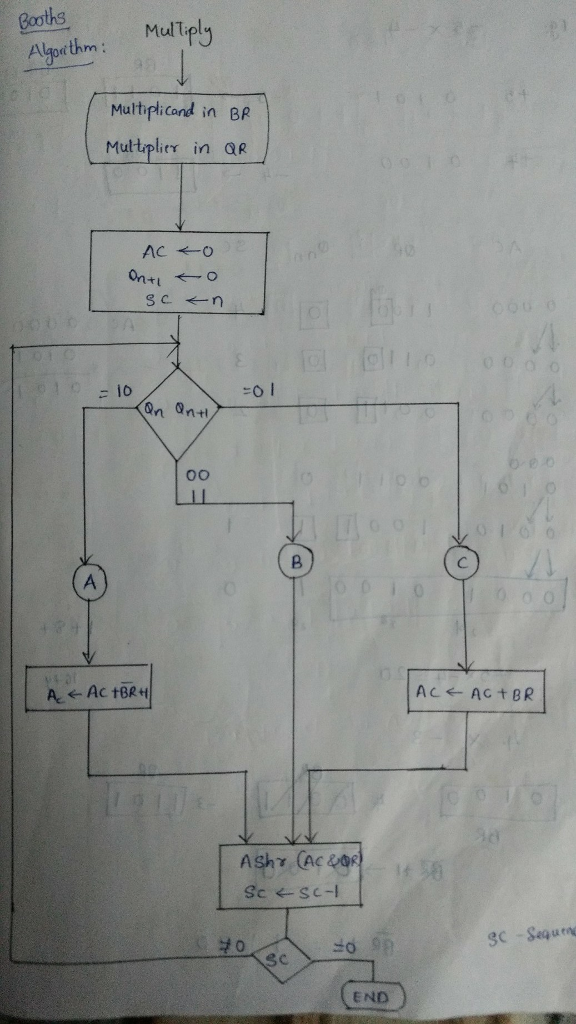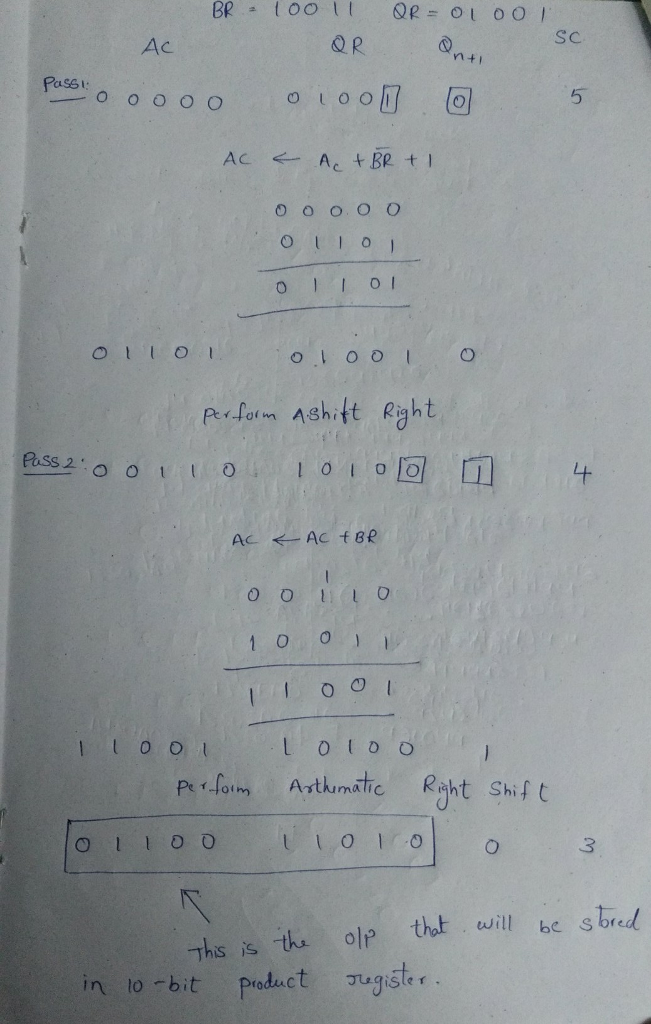# Homework Solution: If the decimal numbers 9 (multiplier) and -13 (multiplicand) were multiplied using Booths algorit…

Booths Algorithm:If the decimal numbers 9 (multiplier) and -13 (multiplicand) were multiplied using Booths algorithm with 5-bit operands, what value would be stored in the 10-bit product register after the second pass?